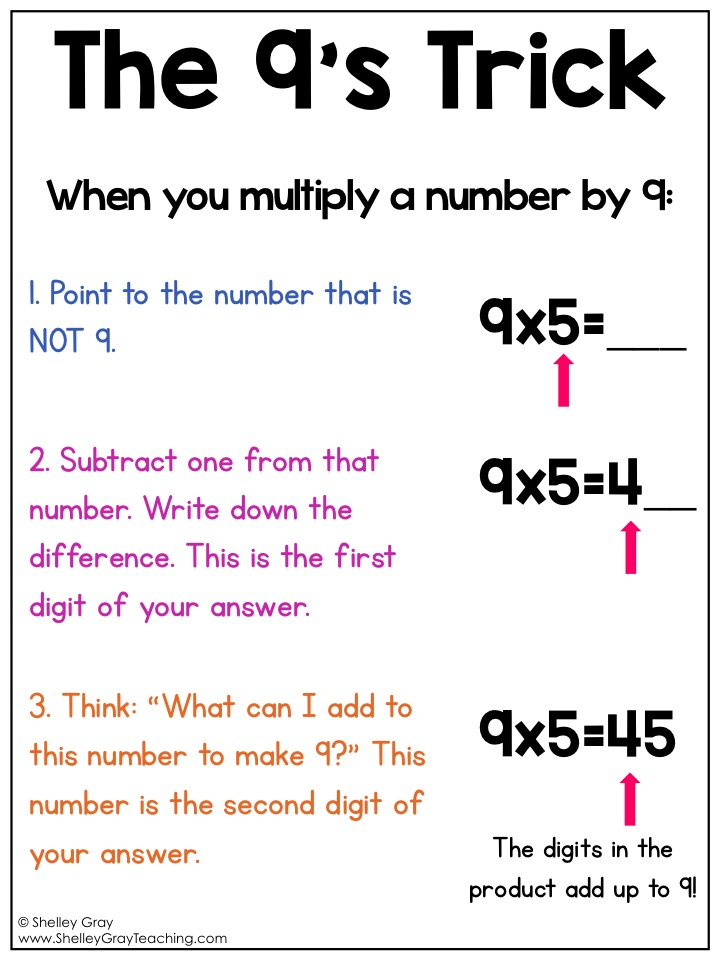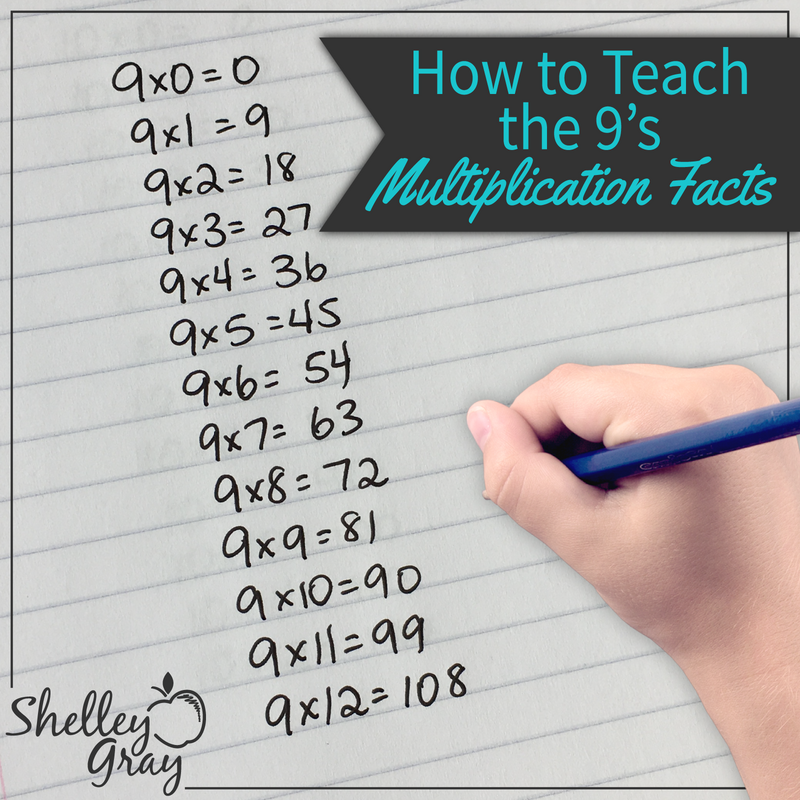# The 9’s Trick for Multiplication {and a freebie}

One of my favorite tricks for multiplication is the 9’s trick. Kids love this too, since it is just so darn neat! I am aware that there are several other popular tricks out there for the 9 times table, but I prefer this one for a couple of reasons. First, it is FAST once you get the hang of it. Second, there is no “finger-counting” involved. As a side note, this trick is only effective for the basic facts (9×10 and less). Here are the written instructions to explain exactly how it works.

Step One: Look at the equation. Point to the number that is NOT the 9. For example, in the equation 9×4=___, point to the 4.

Step Two: Subtract 1 from the number that you are pointing to. In this example, you would think “4-1=3.” The difference (in this case, 3) will be the first number of your product. So now our equation looks like this: 9×4=3__.

Step 3: When you add the numbers in the product together, they will make 9. So now we need to think, “What can I add to 3 to make 9?” In this case the answer is 6. Add this 6 as the second digit in your product: 9×4=36.

And there you have it!

As you can see, this works with any basic multiplication fact involving a 9. Notice that in every case, the sum of the numbers in the product add up to 9!

9×1=9

9×2=18

9×3=27

9×4=36

9×5=45

9×6=54

9×7=63

9×8=72

9×9=81

9×10=90Interested in other strategies for the 9’s multiplication facts? Check out my detailed blog post with lots of pictures HERE.Or register for an upcoming Multiplication and Division Facts for Mastery Webinar HERE. You’ll leave with a ton of new ideas, free resources, a plan of action, a PD certificate, and more!•This was awesome!! I tried it with a struggling student last week. It really works!!! Thanks for sharing Shelley!!! I always knew in my head it was not appropriate to teach the finger trick, but was at a loss for a better way. You found it!!!!

•Love this. Wish I knew about this when I was learning. Can’t wait to use this with my daughter!! Thank you so much.

•I love thew trick ia m testing on my 9’s at school and that trick worked

•This is a good method but involves too many numbers and a (relatively) complex calc which prevents it from replacing memorization. This method is so fast – and it is a calculation. Almost instant because the two ops are on the same number:

Focus ONLY on the multiplier. Count it back one for the 1st digit. Make it into a 10 for the 2nd digit.

For 9 x 4, the 4 counts back to 3 (for thirty- )

then, Make-a-10 out of the 4 for the 2nd digit (six)